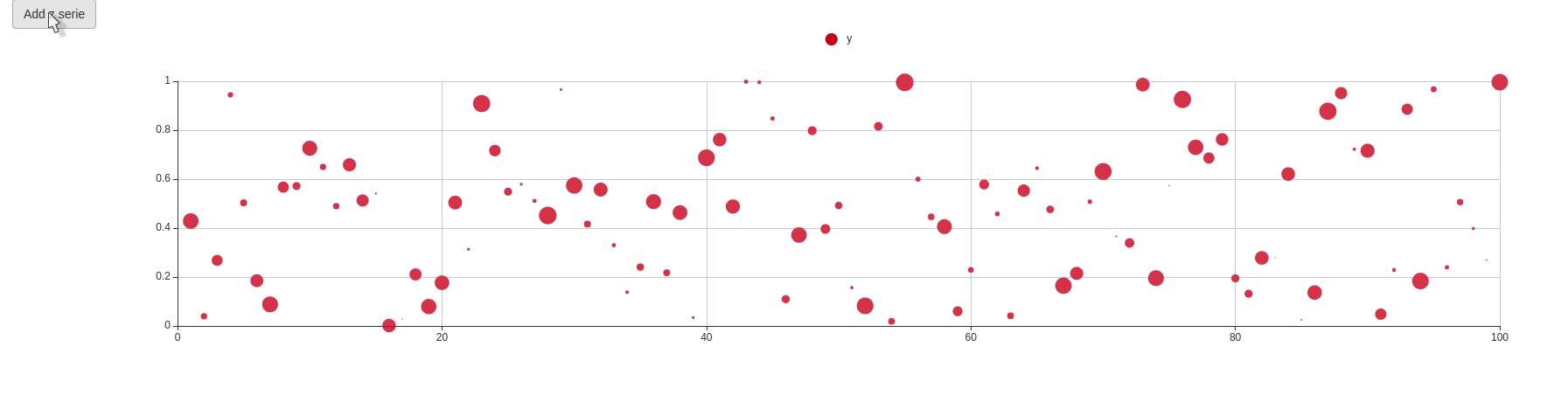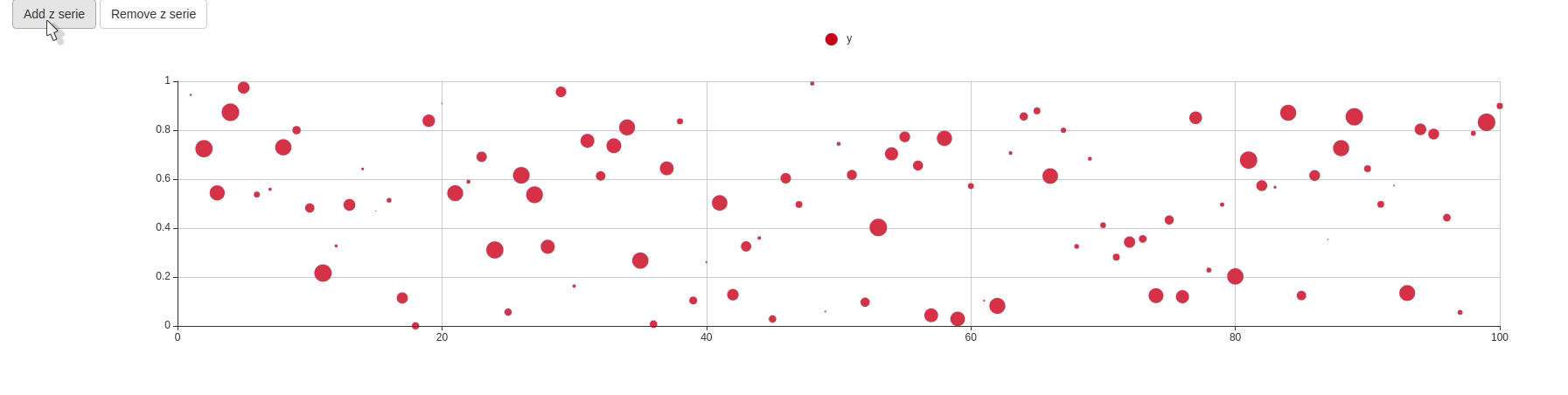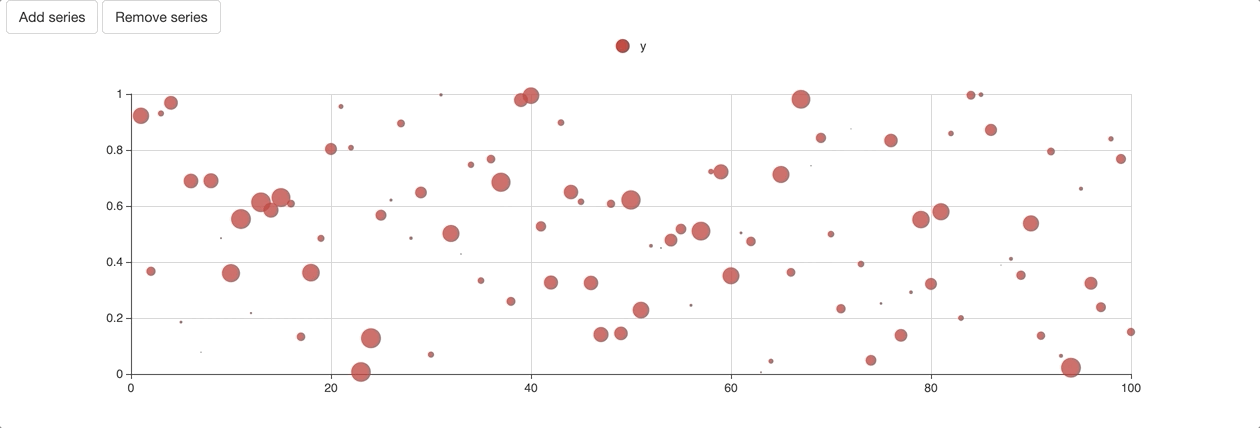Introduction

This showcases some features that are yet to be available on CRAN and currently being tested: feedback in the form of github issues and pull requests is more than welcome!

This essentially covers a consequential new feature of echarts4r that allows using any function that adds a serie to a chart (e.g.: e_bar) as a proxy. This, combined with a new e_remove_serie lets one seamlessly add and remove series from a chart without having to redraw it in its entirety, making for much slicker Shiny charts.

Examples

Below is a basic Shiny application that uses echarts4r. We’ll afterwards add to the app the ability to add and remove a serie.

library(shiny)
library(echarts4r)

# phoney data
df <- data.frame(
x = 1:100,
y = runif(100),
z = runif(100)
)

ui <- fluidPage(
echarts4rOutput("chart")
)

server <- function(input, output){

output$chart <- renderEcharts4r({ e_charts(df, x) %>% e_scatter(y, z) }) } shinyApp(ui, server) We add a button to enable adding a serie on the chart. 1. Create a proxy with echarts4rProxy. 2. We use e_line to add a scatter plot. 3. Actually update the chart with e_execute. First, this means we can now use echarts4rProxy just like our nearly chart initialisation function e_charts. Then we used e_line but you are free to use any other functions that adds a serie to a chart. The e_execute function is used to actually send the the updates to the front end and have the chart updated, this allows batching updates, e.g.: add a scatter plot and a line at once. library(shiny) library(echarts4r) # phoney data df <- data.frame( x = 1:100, y = runif(100), z = runif(100) ) ui <- fluidPage( actionButton("add", "Add z serie"), # button echarts4rOutput("chart") ) server <- function(input, output){ output$chart <- renderEcharts4r({
e_charts(df, x) %>%
e_scatter(y, z)
})

observeEvent(input$add, { echarts4rProxy("chart", data = df, x = x) %>% # create a proxy e_line(z) %>% e_execute() }) } shinyApp(ui, server)We can then add another button to remove the serie with e_remove_serie which does not require the use of e_execute. library(shiny) library(echarts4r) # phoney data df <- data.frame( x = 1:100, y = runif(100), z = runif(100) ) ui <- fluidPage( actionButton("add", "Add z serie"), actionButton("rm", "Remove z serie"), echarts4rOutput("chart") ) server <- function(input, output){ output$chart <- renderEcharts4r({
e_charts(df, x) %>%
e_scatter(y, z)
})

observeEvent(input$add, { echarts4rProxy("chart", data = df, x = x) %>% # create a proxy e_line(z) %>% e_execute() }) observeEvent(input$rm, {
echarts4rProxy("chart") %>%  # create a proxy
e_remove_serie("z")
})

}

shinyApp(ui, server)We can now demonstrate how to add multiple series, and the useful of e_execute.

library(shiny)
library(echarts4r)

# phoney data
df <- data.frame(
x = 1:100,
y = runif(100),
z = runif(100)
)

ui <- fluidPage(
actionButton("rm", "Remove series"),
echarts4rOutput("chart")
)

server <- function(input, output){

output$chart <- renderEcharts4r({ e_charts(df, x) %>% e_scatter(y, z) }) observeEvent(input$add, {
echarts4rProxy("chart", data = df, x = x) %>%  # create a proxy
e_line(z) %>%
e_loess(y ~ x, name = "fit") %>%
e_execute()
})

observeEvent(input\$rm, {
echarts4rProxy("chart") %>%  # create a proxy
e_remove_serie("z") %>%
e_remove_serie("fit")
})

}

shinyApp(ui, server)